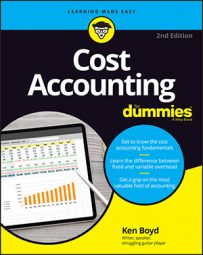##### Cost Accounting For DummiesIn cost accounting, a price variance is the difference between actual and budgeted price for something you purchase. Here’s the formula for price variance:

`Price variance = (Actual price – budgeted price) × (actual quantity)`

An efficiency variance is the difference between actual and budgeted quantities you purchased for a specific price. Here’s the formula for efficiency variance:

`Efficiency variance = (Actual quantity – budgeted quantity) × (standard price or rate)`

A standard is a planned amount per unit. Note that there are two terms in the efficiency formula: standard price and rate. This is a distinction you need to remember for cost accounting. Standard price refers to material variances. You pay a certain price for materials. Standard rate refers to labor variances. When you pay an hourly rate, you’re working with labor variances.

To summarize the distinction between the two variances, you either paid more or less than planned (price variance) or used more or less than planned (efficiency variance).

## How to apply price variances to direct materials in cost accounting

An obvious way to reduce your costs is to analyze the prices you pay for materials. Say you operate a bicycle factory, and you use aluminum to manufacture bike frames. During planning, you come up with a standard or budgeted price of \$5 per pound for aluminum. When you review your actual costs, you find that the real price paid was \$5.75 per pound. Assume you purchased 10,000 pounds of aluminum.

Here is your material price variance for the year.

Material Price Variance — Aluminum for Bikes
Actual Quantity × Actual Price Actual Quantity × Budgeted Price
10,000 lbs. 10,000 lbs.
Multiply by \$5.75 Multiply by \$5.00
Equals \$57,500 (A) Equals \$50,000 (B)
Material price variance (A) – (B) \$7,500

The material price variance is \$7,500 unfavorable because your actual costs (\$57,500) were more than the actual quantity at budgeted price (\$50,000). The only difference in this variance is the price paid for aluminum.

## How to apply efficiency variances in cost accounting

Another way to improve your business performance is to be more efficient — “to work smart,” as the business cliché goes.

During planning, you also determined a budgeted input quantity allowed for actual output of 12,000 pounds of aluminum for bike production. This term multiplies the number of units you plan to produce by some amount of material per unit. This is the calculation for amount of aluminum for the bikes:

```Budgeted input quantity= budgeted units produced × lbs. used in a unit
Budgeted input quantity= 2,000 × 6
Budgeted input quantity= 12,000```

To produce 2,000 bikes, you plan to use 6 pounds of aluminum per bike, or a total of 12,000 pounds. Assume that for the same 2,000 bikes produced, you used only 5 pounds of aluminum per bike (because you were so efficient, of course). This means that you only used 10,000 pounds of aluminum. Your material cost would be lower, which would increase your operating profit.

Here is your material efficiency variance for the year.

Material Efficiency Variance — Aluminum for Bikes
Actual Quantity × Budgeted Price Budgeted Quantity × Budgeted Price
10,000 lbs. 12,000 lbs.
Multiply by \$5.00 Multiply by \$5.00
Equals \$50,000 (A) Equals \$60,000 (B)
Material efficiency variance (A) – (B) (\$10,000)

A negative variance for a cost is favorable, so the material efficiency variance is favorable.

Look back at the price and efficiency variances in the last two tables. Here’s the flexible budget variance calculation for direct materials:

```Flexible budget variance = price variance + efficiency variance
Flexible budget variance = \$7,500 + -\$10,000
Flexible budget variance = -\$2,500```

You have a \$7,500 unfavorable price variance and a \$10,000 favorable efficiency variance. Because this is a cost variance, a negative number indicates less actual spending than planned, and that’s a good thing.

## Lay out reasons for price and efficiency variances in cost accounting

The real reason you go through all of this analysis is to identify areas where you can improve. By “improve,” you want to reduce costs, increase demand, or raise prices to generate a higher profit.

Here are some reasons you might find a material price or efficiency variance:

• Material purchasing decisions: You’re able to negotiate a lower price per unit. You may find a new supplier with a larger supply of material. To reduce their inventory, that supplier may be willing to sell at a lower price. These decisions may create a favorable material variance. On the other hand, a shortage of the material in the marketplace may mean that paying more for materials. The additional spending may create an unfavorable variance.

• Personnel and hiring decisions: Due to a slow economy, you’re can hire workers with the skill set needed, but for a lower hourly rate. Also, you may have staff that’s so productive they get work completed faster than planned. Finally, you’re able to plan production so that workers don’t wait during setup time, which means more hours producing the product. Of course, higher wages, less productivity, and poor decisions may create an unfavorable variance.